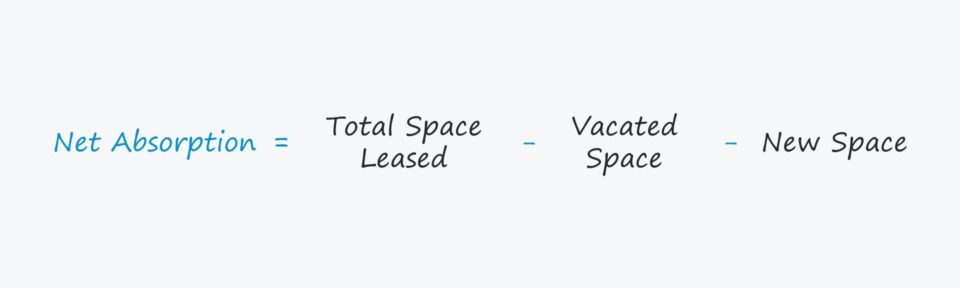Welcome to Wall Street Prep! Use code at checkout for 15% off.# Net Absorption

Guide to Understanding Net Absorption## How to Calculate Net Absorption?

The net absorption metric, in the context of real estate investing, is used to approximate the net change in tenant demand relative to supply.

The net absorption rate is the difference between three metrics tracked over a specified time frame, which are the following:

1. The Occupied Space
2. The Vacated Space (i.e. Unoccupied)
3. The New Space (i.e. New Construction)

The metric is determined by deducting the vacated square footage from the occupied square footage.

In particular, the metric is important to track in the commercial real estate (CRE) market to understand the current state of supply and demand, among other external factors that influence pricing levels.

Calculating net absorption is a three-step process:

1. Determine the Occupied Square Footage (i.e. Leased Space)
2. Calculate the Unoccupied Square Footage (i.e. Vacant Space)
3. Subtract the Unoccupied Square Footage and New Space from the Occupied Square Footage

## Net Absorption Formula

The formula for calculating net absorption is as follows.

Net Absorption = Total Space LeasedVacated SpaceNew Space

From the total leased space, the sum of square feet that became physically vacant and new space (i.e. new construction) are subtracted.

The figures in the formula are typically denoted in units of square feet (sq. ft).

## Gross Absorption vs. Net Absorption: What is the Difference?

The concept of absorption in real estate is a method used to gauge tenant demand in the current market. Most often, absorption is measured in units of square footage (sq. ft).

The two primary absorption metrics are calculated on a gross and net basis:

1. Gross Absorption → The total new square footage leased by tenants over a specific period.
2. Net Absorption → The total new square footage leased by tenants, minus the square footage no longer occupied over a specific period.

While the former represents the total amount of leased space, the latter captures the net change in demand relative to the supply in the market.

## Positive vs. Negative Net Absorption: What is the Difference?

In the real estate market, tracking the net absorption provides useful insights regarding the current state of the market, especially in terms of understand the market demand at present.

• Positive Net Absorption → A greater amount of space was leased compared to the amount that became available on the market, reflecting a decline in the supply of commercial space available. Given a positive rate, lease prices in the market are more likely to increase, which can attract more real estate investors and developers to enter the market and more actively build new spaces to meet tenant demand.
• Negative Net Absorption → A greater amount of space has become vacant and is now available on the market compared to the occupied amount, indicating the demand for commercial real estate spaces has declined relative to the supply. Contrary to the above, the reverse effects are observed given a negative rate, as investors and developers are less likely to engage in the market in such a case since lease prices are lower (and less profitable).20+ Hours of Online Video Training

### Master Real Estate Financial Modeling

This program breaks down everything you need to build and interpret real estate finance models. Used at the world's leading real estate private equity firms and academic institutions.